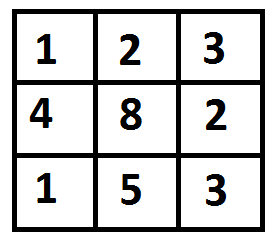GFG App
Open AppBrowser
Continue

# Java Program for Min Cost Path

Given a cost matrix cost[][] and a position (m, n) in cost[][], write a function that returns cost of minimum cost path to reach (m, n) from (0, 0). Each cell of the matrix represents a cost to traverse through that cell. Total cost of a path to reach (m, n) is sum of all the costs on that path (including both source and destination). You can only traverse down, right and diagonally lower cells from a given cell, i.e., from a given cell (i, j), cells (i+1, j), (i, j+1) and (i+1, j+1) can be traversed. You may assume that all costs are positive integers.

For example, in the following figure, what is the minimum cost path to (2, 2)?The path with minimum cost is highlighted in the following figure. The path is (0, 0) –> (0, 1) –> (1, 2) –> (2, 2). The cost of the path is 8 (1 + 2 + 2 + 3).

## Java

 `/* Java program for Dynamic Programming implementation ` `of Min Cost Path problem */` `import` `java.util.*; `   `class` `MinimumCostPath { ` `    ``/* A utility function that returns minimum of 3 integers */` `    ``private` `static` `int` `min(``int` `x, ``int` `y, ``int` `z) ` `    ``{ ` `        ``if` `(x < y) ` `            ``return` `(x < z) ? x : z; ` `        ``else` `            ``return` `(y < z) ? y : z; ` `    ``} `   `    ``private` `static` `int` `minCost(``int` `cost[][], ``int` `m, ``int` `n) ` `    ``{ ` `        ``int` `i, j; ` `        ``int` `tc[][] = ``new` `int``[m + ``1``][n + ``1``]; `   `        ``tc[``0``][``0``] = cost[``0``][``0``]; `   `        ``/* Initialize first column of total cost(tc) array */` `        ``for` `(i = ``1``; i <= m; i++) ` `            ``tc[i][``0``] = tc[i - ``1``][``0``] + cost[i][``0``]; `   `        ``/* Initialize first row of tc array */` `        ``for` `(j = ``1``; j <= n; j++) ` `            ``tc[``0``][j] = tc[``0``][j - ``1``] + cost[``0``][j]; `   `        ``/* Construct rest of the tc array */` `        ``for` `(i = ``1``; i <= m; i++) ` `            ``for` `(j = ``1``; j <= n; j++) ` `                ``tc[i][j] = min(tc[i - ``1``][j - ``1``], ` `                            ``tc[i - ``1``][j], ` `                            ``tc[i][j - ``1``]) ` `                        ``+ cost[i][j]; `   `        ``return` `tc[m][n]; ` `    ``} `   `    ``/* Driver program to test above functions */` `    ``public` `static` `void` `main(String args[]) ` `    ``{ ` `        ``int` `cost[][] = { { ``1``, ``2``, ``3` `}, ` `                        ``{ ``4``, ``8``, ``2` `}, ` `                        ``{ ``1``, ``5``, ``3` `} }; ` `        ``System.out.println(``"minimum cost to reach"` `+ ` `                    ``" (2, 2) = "` `+ minCost(cost, ``2``, ``2``)); ` `    ``} ` `} ` `// This code is contributed by Pankaj Kumar `

Output:

`minimum cost to reach (2, 2) = 8`

Time Complexity: O(m*n)

Auxiliary Space: O(m*n)

Please refer complete article on Dynamic Programming | Set 6 (Min Cost Path) for more details!

My Personal Notes arrow_drop_up